# Bonuses

Five employees of the company were paid bonuses so that each successor received a 550 USD less than the previous employee. How much did everyone get, if a total of USD 11,000 has paid?

Result

a =  3300
b =  2750
c =  2200
d =  1650
f =  1100

#### Solution:

a+b+c+d+f = 11000
b = a-550
c = b-550
d = c-550
f = d-550

a+b+c+d+f = 11000
a-b = 550
b-c = 550
c-d = 550
d-f = 550

a = 3300
b = 2750
c = 2200
d = 1650
f = 1100

Calculated by our linear equations calculator.

Leave us a comment of example and its solution (i.e. if it is still somewhat unclear...):Be the first to comment!#### To solve this example are needed these knowledge from mathematics:

Looking for help with calculating arithmetic mean? Looking for a statistical calculator? Do you have a linear equation or system of equations and looking for its solution? Or do you have quadratic equation?

## Next similar examples:

1. SeatsSeats in the sport hall are organized so that each subsequent row has five more seats. First has 10 seats. How many seats are: a) in the eighth row b) in the eighteenth row
2. The sum 2The sum of five consecutive even integers is 150. Find the largest of the five integers. A.28 B.30 C.34 D.54 Show your solution and explain your answer.
3. Variance and average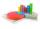Of the 40 values were calculated average mx = 7.5 and variance sx = 2.25. After the control was found to lack the two items of the values of x41 = 3.8 and x42=7. Correct the above characteristics (mx and sx).
4. Crystal water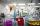The chemist wanted to check the content of water of crystallization of chromic potassium alum K2SO4 * Cr2 (SO4) 3 * 24 H2O, which was a long time in the laboratory. From 96.8 g of K2SO4 * Cr2 (SO4) 3 * 24 H2O prepared 979 cm3 solution of base. S
5. SequenceBetween numbers 1 and 53 insert n members of the arithmetic sequence that its sum is 702.
6. Median and modusRadka made 50 throws with a dice. The table saw fit individual dice's wall frequency: Wall Number: 1 2 3 4 5 6 frequency: 8 7 5 11 6 13 Calculate the modus and median of the wall numbers that Radka fell.
7. Third memberDetermine the third member of the AP if a4=93, d=7.5.
8. 75th percentile (quartille Q3)Find 75th percentile for 30,42,42,46,46,46,50,50,54
9. Sequence 3Write the first 5 members of an arithmetic sequence: a4=-35, a11=-105.
10. Difference AP 4Calculate the difference of the AP if a1 = 0.5, a2 + a3 = -1.1
11. AS sequenceIn an arithmetic sequence is given the difference d = -3 and a71 = 455. a) Determine the value of a62 b) Determine the sum of 71 members.
12. Theorem proveWe want to prove the sentence: If the natural number n is divisible by six, then n is divisible by three. From what assumption we started?
13. Difference AP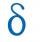Calculate the difference of arithmetic progression if the sum of its first 19 members Sn = 8075 and the first member is a1 = 20
14. Determine AP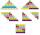Determine the difference of the arithmetic progression if a3 = 7, and a4 + a5 = 71
15. Fifth memberDetermine the fifth member of the arithmetic progression, if the sum of the second and fifth members equal to 73, and difference d = 7.
16. Evaluation of expressionsIf a2-3a+1=0, find (i)a2+1/a2 (ii) a3+1/a3
17. Series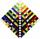Your task is express the sum of the following arithmetic series for n = 14: S(n) = 11 + 13 + 15 + 17 + ... + 2n+9 + 2n+11